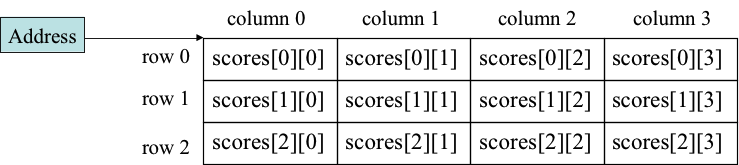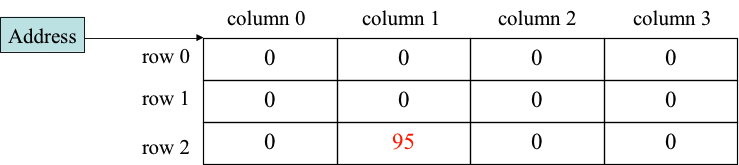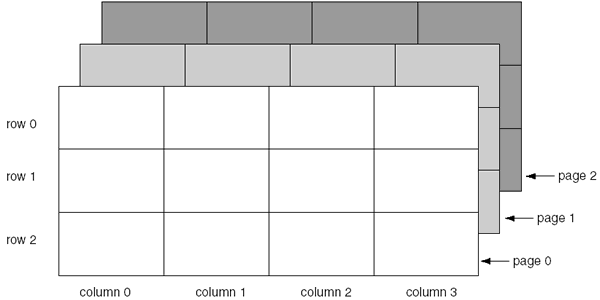# 2D Arrays, Sorting, and Binary Search

1. Contents
2. Two-Dimensional Arrays
3. Some Sorting Algorithms
4. Binary Search

## Two-Dimensional Arrays

### Introduction to Two-Dimensional Arrays

• A two-dimensional array is an array of arrays.

• It can be thought of as having rows and columns.

• Declaring a two-dimensional array requires two sets of brackets and two size declarators.

• The first one is for the number of rows.

• The second one is for the number of columns.

• Example:

```int[][] scores = new int;
// number of rows:    3
// number of columns: 4```
• The two sets of brackets in the data type indicate that the scores variable will reference a two-dimensional array.

• Notice that each size declarator is enclosed in its own set of brackets.

### Accessing Two-Dimensional Array Elements

• When processing the data in a two-dimensional array, each element has two subscripts:

• One for its row
• The other for its column
• The `scores` variable holds the address of a two-dimensional array of integers.• Accessing one of the elements in a two-dimensional array requires the use of both subscripts.

`scores == 95`• Programs that process two-dimensional arrays can do so with nested loops.

• Book example:

• Example code:

```import java.util.Scanner;

public class Program {
public static void main(final String[] args) {

// Initialize a 2D array of Strings.
final String[][] array = new String;

// Prompt the user to populate the 2D array.
final Scanner scanner = new Scanner(System.in);
for (int row = 0; row < array.length; row++) {
for (int col = 0; col < array[row].length; col++) {
System.out.print("scores"
+ "[" + row + "]"
+ "[" + col + "]: ");
System.out.flush();

array[row][col] = scanner.nextLine();
}
}

// Print the contents of the 2D array.
System.out.println();
for (final String[] row : array) {
for (final String col : row)
System.out.print(col + "\t");
System.out.println();
}
}
}```

### Initializing a Two-Dimensional Array

• Initializing a two-dimensional array requires enclosing each row's initialization list in its own set of braces.

```int[][] nums = {{1, 2}, {3 ,4}, {5, 6}};
int[][] nums = {{1, 2}, {3 ,4}, {5, 6, 7}};```
• Example code:

```public class Program {
public static void main(final String[] args) {

// Initialize 2D arrays of integers.
final int[][] array = {
{ 1, 2 },
{ 3, 4 },
{ 5, 6, 7, 8 }
};

// Print the contents of the 2D arrays.
for (final int[] row : array) {
for (final int col : row)
System.out.print(col + "\t");
System.out.println();
}
}
}```

### The `length` Field of Two-Dimensional Arrays

• 2D arrays are arrays of 1D arrays.

• The length field of the array gives the number of rows in the array.

• Each row has a length constant that tells how many columns are in that row.

• Each row can have a different number of columns.

• Book example:

• Example code:

### Ragged Arrays

• When the rows of a 2D array are of different length, the array is known as a ragged array.

• We can create a ragged array by creating a 2D array with a specific number of rows, but no columns. Then create the individual rows.

`int[][] ragged = new int[]`
• Example code:

```public class Program {
public static void main(final String[] args) {

// Initialize 2D arrays of integers.
final int[][] array = new int[];
array = new int[] { 1, 2, 3 };
array = new int[] { 1, 2, 3, 4 };
array = new int[] { 1, 2, 3, 5, 6 };

// Print the contents of the 2D arrays.
for (final int[] row : array) {
for (final int col : row)
System.out.print(col + "\t");
System.out.println();
}
}
}```

### More Than Two Dimensions

• Java does not limit the number of dimensions that an array may be.

• More than three dimensions is hard to visualize, but can be useful in some programming problems.• Example code:

```public class Program {
public static void main(final String[] args) {

// Initialize 2D arrays of integers.
final int[][][] array = {
{ { 1,  2 }, {  3,  4 } },
{ { 5,  6 }, {  7,  8 } },
{ { 9, 10 }, { 11, 12 } }
};

// Print the contents of the 2D arrays.
for (final int[][] page : array) {
for (final int[] row : page) {
for (final int col : row)
System.out.print(col + "\t");
System.out.println();
}
System.out.println();
System.out.println();
}
}
}```

## Some Sorting Algorithms

There are many sorting algorithms. Most of them are more complex but run faster than the ones introduced below. Quick sort, heap sort, and merge sort are such examples. For detailed information about more complex sorting algorithms, visit sorting-algorithms.com

### Selection Sort

• Find the smallest or largest element in the array, and put it in the proper place, such as index `0`. Repeat this procedure until the array is sorted.

• Book example:

• A visual demonstration of selection sort

• Example code:

```public class Program {

public static void main(final String[] args) {
final int[] array = { 5, 2, 6, 7, 12, 1, 0 };

selectionSort(array);

for (final int item : array)
System.out.print(item + " ");
}

private static void selectionSort(final int[] array) {
// Loop over the entire array.
for (int i = 0; i < array.length; i++) {

// Find the next element with the smallest
// value.
int min = i;
for (int j = i + 1; j < array.length; j++)
if (array[j] < array[min])
min = j;

// If any element had a smaller value,
// then swap it.
if (min != i) {
final int temp = array[i];
array[i] = array[min];
array[min] = temp;
}
}
}
}```

### Insertion Sort

• Take an element from the unsorted part of the array and insert the item in the proper place of the sorted part of the array.

• A visual demonstration of insertion sort

• Example code:

```public class Program {
public static void main(final String[] args) {
final int[] array = { 5, 2, 6, 7, 12, 1, 0 };

insertionSort(array);

for (final int item : array)
System.out.print(item + " ");
}

private static void insertionSort(final int[] array) {

// Loop over the entire array.
for (int i = 1; i < array.length; i++) {

// Loop backward from i to find where
// the ith element should be inserted,
// swapping elements along the way.
final int value = array[i];
int j = i - 1;
while (j >= 0 && array[j] > value)
array[j + 1] = array[j--];

// Insert the ith element in order.
array[j + 1] = value;
}
}
}```

### Bubble Sort

• Exchange two adjacent elements if they are out of order. Repeat until the array is sorted.

• A visual demonstration of bubble sort

• Example code:

```public class Program {
public static void main(final String[] args) {
final int[] array = { 5, 2, 6, 7, 12, 1, 0 };

bubbleSort(array);

for (final int item : array)
System.out.print(item + " ");
}

private static void bubbleSort(final int[] array) {

// Loop until no elements are out
// of order.
boolean isSwapped;
do {
isSwapped = false;

// Loop over the entire array.
for (int i = 0; i < array.length - 1; i++) {

// are out of order, swap them.
if (array[i] > array[i + 1]) {
final int temp = array[i];
array[i] = array[i + 1];
array[i + 1] = temp;
isSwapped = true;
}
}

} while (isSwapped);
}
}```

## Binary Search

• A binary search requires an array that is already sorted.

• The procedure:

• A binary search starts with the element in the middle of the array.

• If that element is the desired value, the search is over.

• Otherwise, the value in the middle element is either greater or less than the desired value.

• If it is greater than the desired value, search in the first half of the array.

• Otherwise search the last half of the array.

• Repeat as needed while adjusting the start and end points of the search.

• Book example:

• Example code:

```public class Program {
public static void main(final String[] args) {
final int[] array = { 0, 1, 2, 5, 12, 17, 30 };

final int value = 12;
final int index = binarySearch(array, value);

System.out.println(
value
+ " is at index: "
+ index);
}

private static int binarySearch(
final int[] array,
final int value) {

// Store the initial range of the search.
int start = 0;
int end = array.length - 1;

// Loop until the range is zero.
while (start <= end) {

// Find the mid-point in the range.
int middle = (start + end) / 2;

// If the value is found at the
// mid-point, return it.
if (array[middle] == value)
return middle;

// Otherwise, cut the range in half
// before looping.
if (array[middle] < value)
start = middle + 1;
else
end = middle - 1;
}

// Return -1 to indicate the